Isometric Tiles Introduction

This beginners tutorial is for those curious about making a game using isometric tiles. We'll cover the very basics of what isometric tiles are, why they're useful, and basic decisions to make when choosing isometric tiles.

Common Projections

The visual world around us is three dimensional, but the games we play (for now) are on a 2D screen. "Projection" is, in simple terms, the way we "flatten" a 3D view into 2D.Three common projections of the same object

There are several popular projections used in 2D games. The most popular by far is to have the camera exactly on a major axis. This is common in puzzle games and side scrollers, where each tile is a simple square and the third dimension isn't visible at all. This view is often directly overhead, or directly from one side. If we look at a cube in this view, only one side would be visible (just the facing side).

Side note: I highly recommend making a game or tile set using this simple projection before moving up to isometric! Basically everything that applies to a flat 2D game also applied to isometric, but isometric adds an extra layer of calculations.

The next most common projection still uses square shaped tiles, but changes the angle of the camera so we can see the third dimension. Games using this projection add movement in that third dimension. We see this projection common in early console-style RPGs and side scrolling beat-em-ups. Our virtual "camera" is angled in one direction to get this view. If we look at a cube in this view, two sides are visible (the top and facing side).

For Isometric Projection we angle our camera along two axes (swing the camera 45 degrees to one side, then 30 degrees down). This creates a diamond (rhombus) shaped grid where the grid spaces are twice as wide as they are tall. This style was popularized by strategy games and action RPGs. If we look at a cube in this view, three sides are visible (top and two facing sides).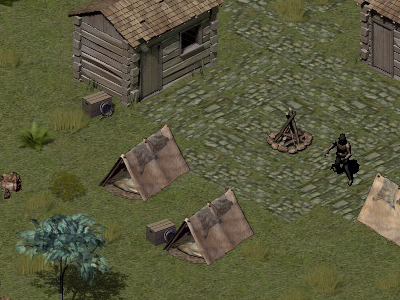Flare
: isometric view

There are many other less-common projection styles available for 2D games. The grid can be at a 45 degree angle but use "square" grid spaces as seen in Ultima Online. Or the grid can be square and 90 degree angles, with walls rising at 45 degrees as seen in Ultima 7. Or the camera can be turned so that each axis is affected differently as in the original Fallout games (which actually use a hex grid).

Isometric Specifics

In true isometric projection, grid lines are all at 30 degrees and each segment represents the same length -- making it useful in engineering diagrams.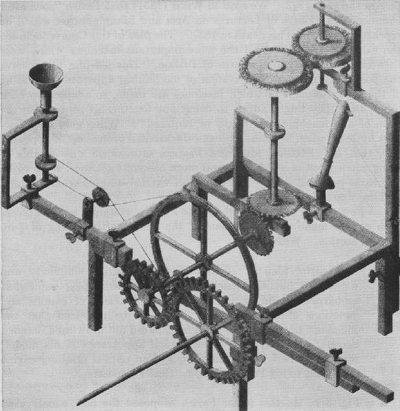Isometric engineering diagram from 1822

In video games when we say "isometric" we usually mean a view that's not exactly isometric. Video game isometric prefers a slightly different angle because we're working with whole pixels.

The following diagram shows several lines drawn in pixels (zoomed in to see details). Notice how the green lines look nice while the red lines look jagged. This is because the green lines are using specific slopes that fit exactly on a pixel grid. Our isometric line is the 1:2 slope -- draw two pixels horizontally for every one pixel vertically. This means each isometric grid space is exactly twice as wide as it is tall (see the blue isometric outline).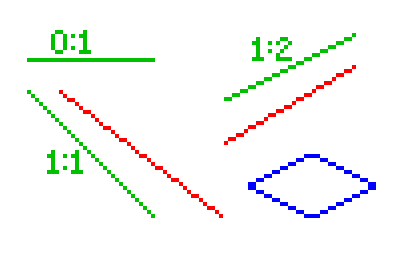Nice vs. jagged pixel lines

Because of this predictable 1:2 slope it is easy to create pixel art in isometric style. It's also easy to render 3D art in isometric projection. In Blender we can use an orthographic camera to create isometric art:

• Camera angle (60, 0, 45) for video-game style isometric (tiles that are 2x wide as they are tall)
• Camera angle (54.736, 0, 45) for true engineering isometric (but jagged edges due to the angles)

The pseudo-isometric projection not only makes pixel art crisp, but makes map coordinates easy to handle. Every grid space is exactly 2x wide as it is tall, so calculating the screen position of a particular grid space is straight forward.

Choosing a Tile Size

Generally a game will use the same base tile size for the entire project. So choosing the right size from the beginning is an important task.

First there's the pixel dimensions of the tile. In all video game art it's common to stick with powers-of-two dimensions for images. So the most common grid size of isometric games are 32x16, or 64x32, or 128x64. Note that it's not really necessary to use a power of two. You might decide 100x50 is easier to work with. Sticking with powers of two might allow you to do nifty computing tricks (bit shifting instead of multiplying/dividing). If you plan to make tiles that are reusable in other projects/games, it's probably smart to stick with the traditional sizes.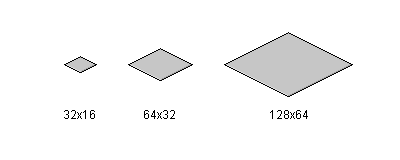Common tile sizes

• 32x16 is a very small size but still useful on mobile devices or if using scaled-up pixel art.
• 64x32 is a common modern tile size, flexible for many game types.
• 128x64 is good for games with a high level of detail or displayed on HD resolutions.

Even if you choose e.g. 64x32 base grid size, that doesn't mean every image in your game will be 64px by 32px. It's common in isometric games to have tall tiles that align with the bottom of the grid. A game using 64x32 might actually use 64px x 128px images for objects such as walls -- or rather, each section of wall that fits exactly on one grid space. Using tall tiles is useful for drawing objects in the correct z-order. But more on that another time.

Once you choose a tile size, you still need to think about what that tile size represents in 3D space: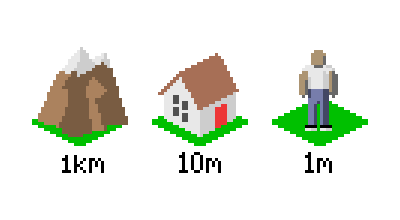Example tile scales

• If you're making a world conquest turn-based strategy, each tile might represent a 1km square area or more.
• If you're making a city building simulation, one tile might be a 10m x 10m square. This could be the width of a city street or size of a small building.
• If you're making an RPG where the focus is on one hero, each tile might represent 1m square.

This really depends on the genre of game and on the target display size. I suggest mocking up screens of your game to get a feel for what size and scale will work best. Then, choose a scale and stick with it. It will be easier to create matching assets when you know exactly what size each tile represents.

That's it for our introduction. In the next part of this series we'll look at approaches to making floor and wall tiles. If you have questions about isometric tiles that you'd like me to answer during this series, please drop me a message!Clint Bellanger is a software developer who has been experimenting with video game code for 20 years and 3D art for 10 years. His latest project is Flare, a Free/Libre action roleplaying engine.